## RS Aggarwal Solutions Class 10 Chapter 19 Volume and Surface Areas of Solids MCQ

These Solutions are part of RS Aggarwal Solutions Class 10. Here we have given RS Aggarwal Solutions Class 10 Chapter 19 Volume and Surface Areas of Solids MCQ.

Other Exercises

Question 1.
Solution:
Correct option: (a)
A cylindrical pencil sharpened at one edge is the combination of a cylinder and a cone. Observe the figure, the lower portion is a cylinder and the upper tapering portion is a cone.

Question 2.
Solution:
Correct option: (b)
A shuttlecock used for playing badminton is the combination of a frustum of a cone and a hemisphere,the lower portion being the hemisphere and the portion above that being the frustum of the cone.

Question 3.
Solution:
Correct option: (c)
A funnel is the combination of a cylinder and frustum of a cone. The lower portion is cylindrical and the upper portion is a frustum of a cone.

Question 4.
Solution:
Correct option: (a)
A surahi is a combination of a sphere and a cylinder, the lower portion is the sphere and the upper portion is the cylinder.

Question 5.
Solution:
Correct option: (b)
The shape of a glass (tumbler) is usually in the form of a frustum of a cone.

Question 6.
Solution:
Correct option: (c)
The shape of a gill in the gilli-danda game is a combination of two cones and a cylinder. The cones at either ends with the cylinder in the middle.

Question 7.
Solution:
Correct option: (a)
A plumbline (sahul) is the combination of a hemisphere and a cone, the hemisphere being on top and the lower portion being the cone.

Question 8.
Solution:
Correct option: (d)
A cone is cut by a plane parallel to its base and the upper part is removed. The part that is left over is called the frustum of a cone.

Question 9.
Solution:
Correct option: (c)
During conversion of a solid from one shape to another, the volume of the new shape will remain altered.

Question 10.
Solution:
Correct option: (c)
In a right circular cone, the cross section made by a plane parallel to the base is a circle.

Question 11.
Solution: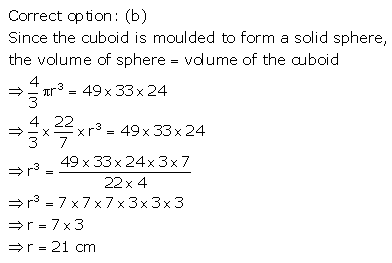Question 12.
Solution: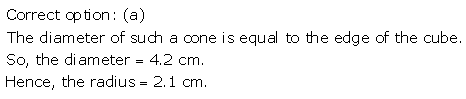Question 13.
Solution:Question 14.
Solution: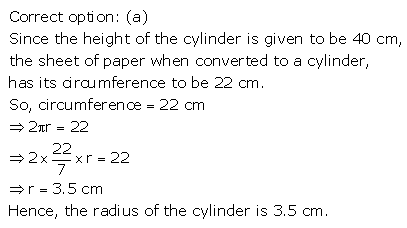Question 15.
Solution: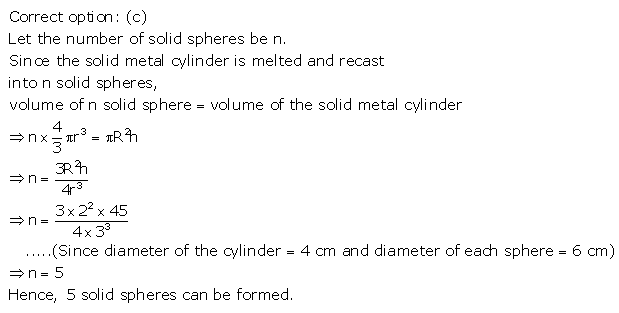Question 16.
Solution: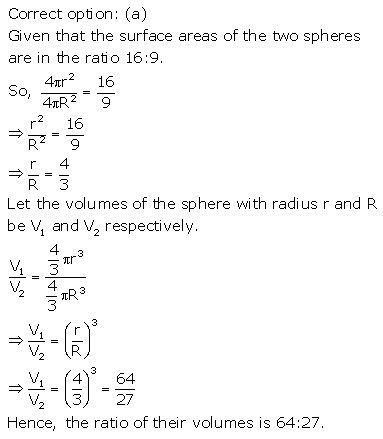Question 17.
Solution: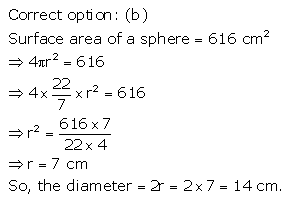Question 18.
Solution: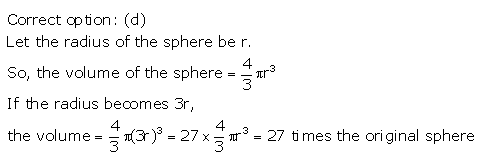Question 19.
Solution: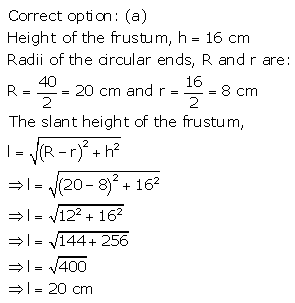Question 20.
Solution:Question 21.
Solution: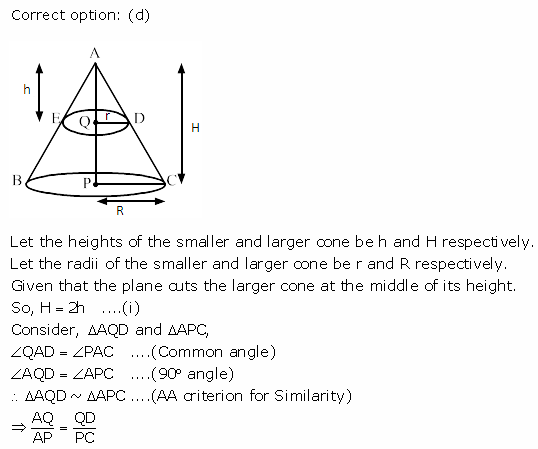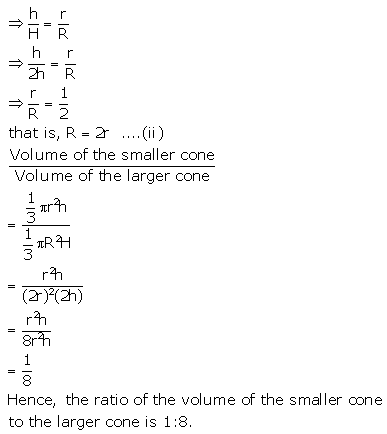Question 22.
Solution: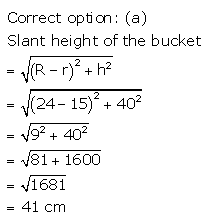Question 23.
Solution:Question 24.
Solution: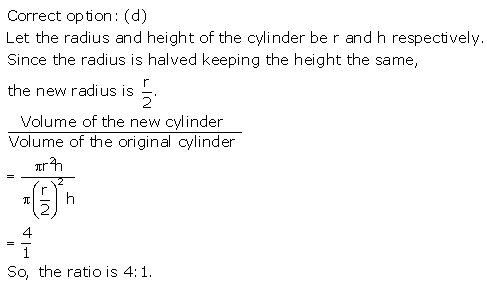Question 25.
Solution:Question 26.
Solution:Question 27.
Solution:Question 28.
Solution: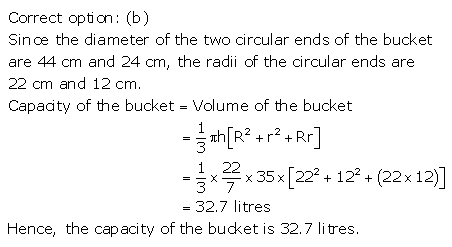Question 29.
Solution: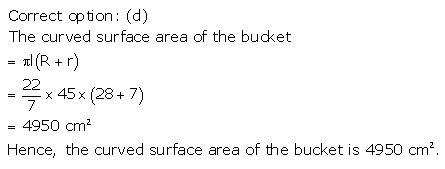Question 30.
Solution: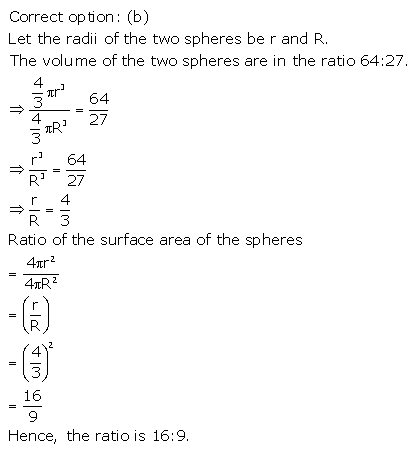Question 31.
Solution:Question 32.
Solution: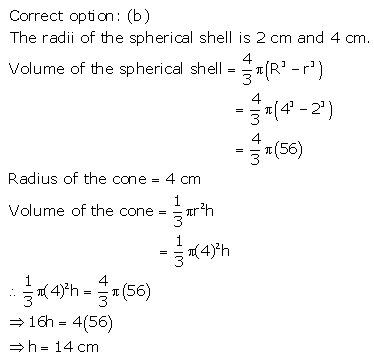Question 33.
Solution: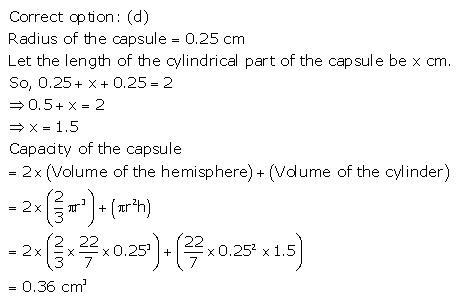Question 34.
Solution: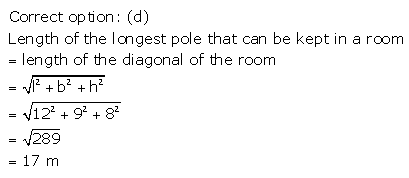Question 35.
Solution: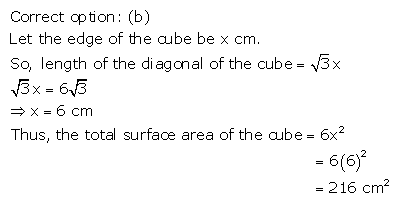Question 36.
Solution: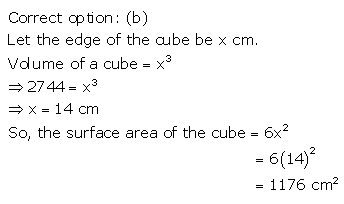Question 37.
Solution: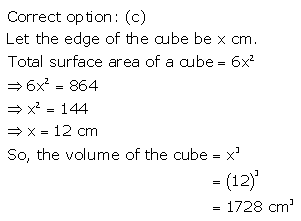Question 38.
Solution:Question 39.
Solution: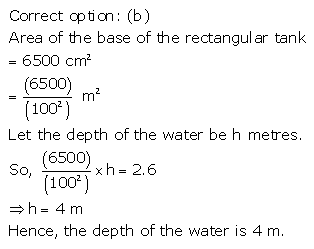Question 40.
Solution: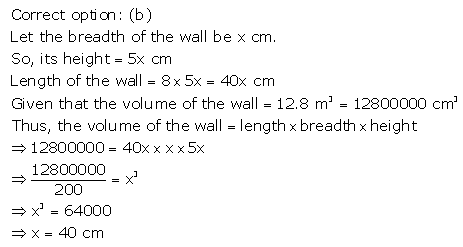Question 41.
Solution:Question 42.
Solution: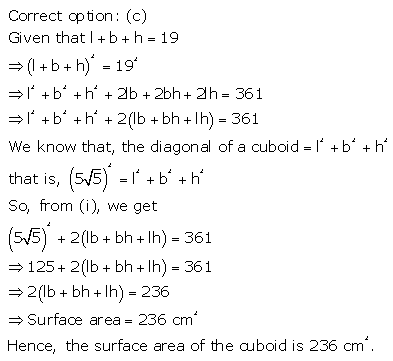Question 43.
Solution: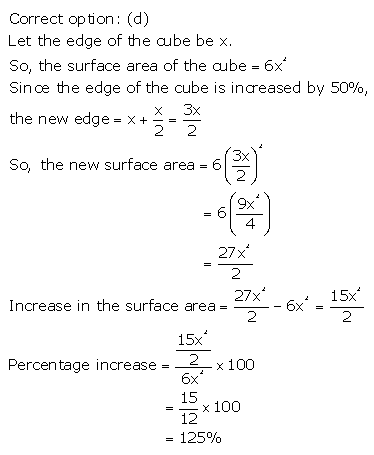Question 44.
Solution: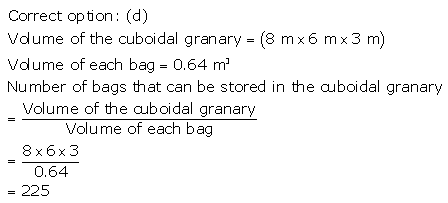Question 45.
Solution: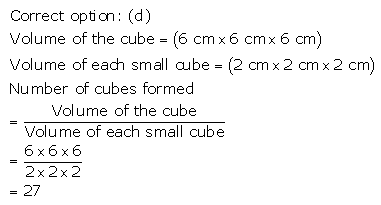Question 46.
Solution:Question 47.
Solution:Question 48.
Solution: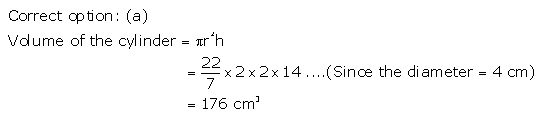Question 49.
Solution: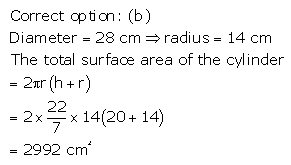Question 50.
Solution: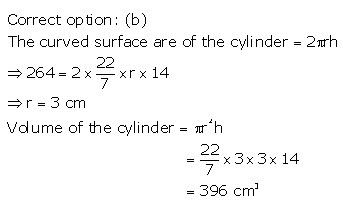Question 51.
Solution: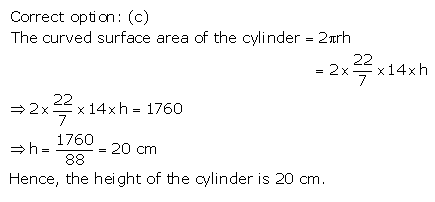Question 52.
Solution:Question 53.
Solution: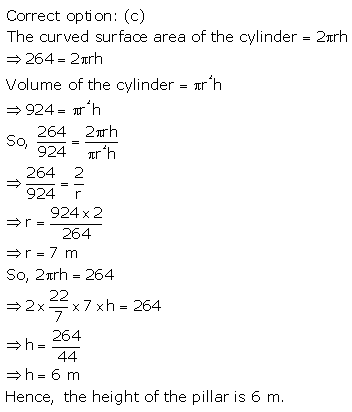Question 54.
Solution: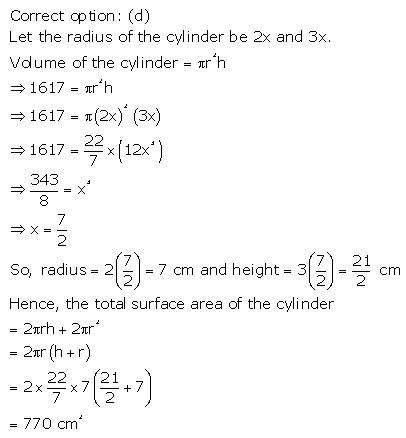Question 55.
Solution: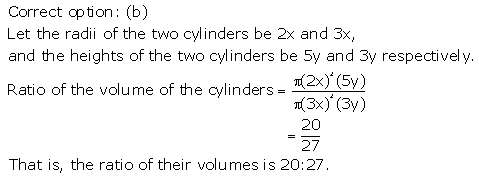Question 56.
Solution:Question 57.
Solution: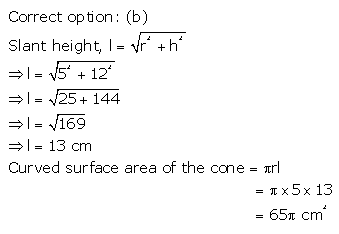Question 58.
Solution: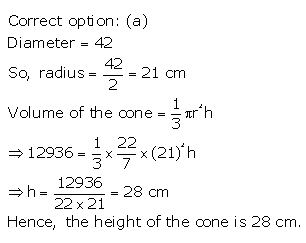Question 59.
Solution: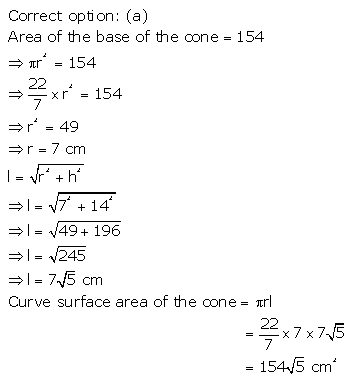Question 60.
Solution: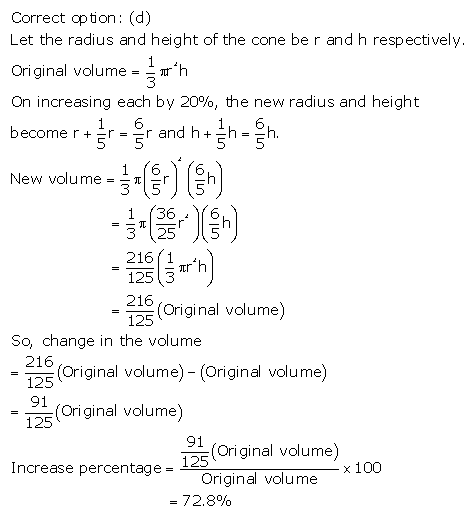Question 61.
Solution: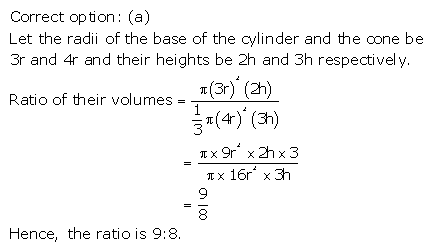Question 62.
Solution: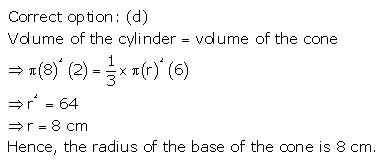Question 63.
Solution: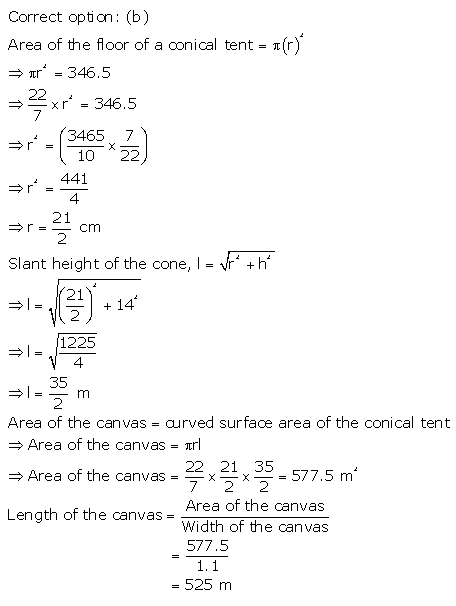Question 64.
Solution:Question 65.
Solution: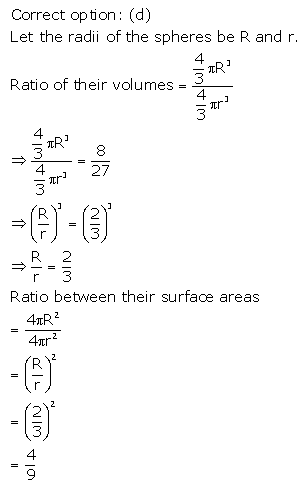Question 66.
Solution:Question 68.
Solution: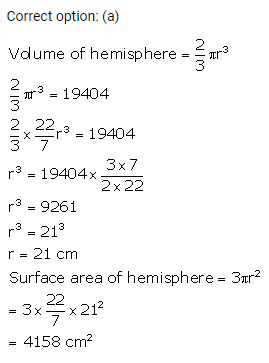Question 69.
Solution: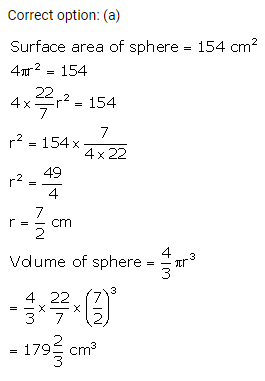Question 70.
Solution: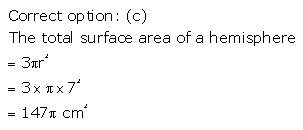Question 71.
Solution: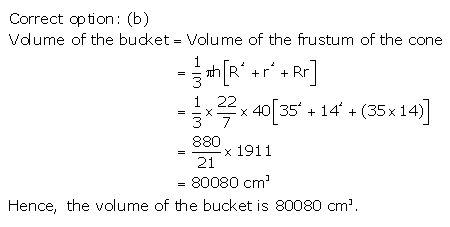Question 72.
Solution:Question 73.
Solution: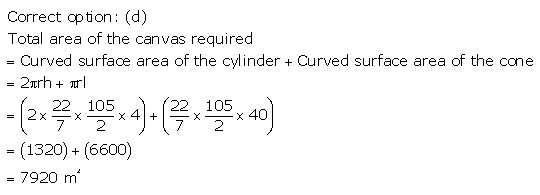Question 74.
Solution:Question 75.
Solution: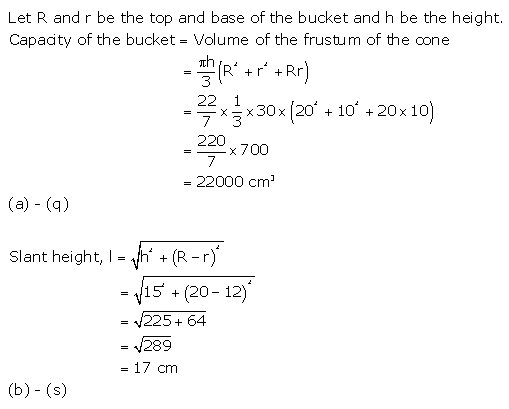Question 76.
Solution:Question 77.
Solution:Question 78.
Solution:Question 79.
Solution: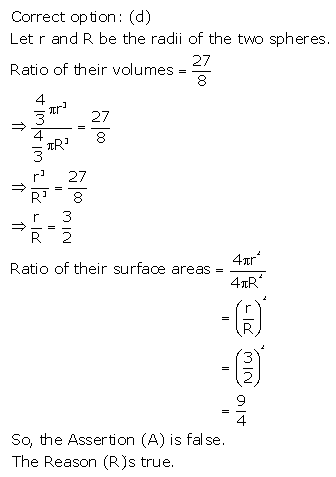Question 80.
Solution: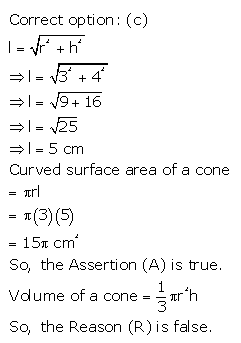We hope the RS Aggarwal Solutions Class 10 Chapter 19 Volume and Surface Areas of Solids MCQ help you. If you have any query regarding RS Aggarwal Solutions Class 10 Chapter 19 Volume and Surface Areas of Solids MCQ, drop a comment below and we will get back to you at the earliest.